##全国校区# 基于OpenCV的图像卡通化2020-08-11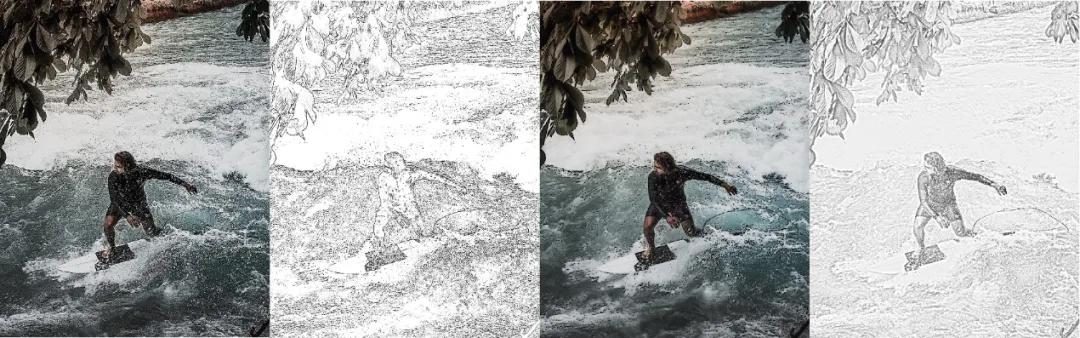1. 铅笔素描

2. 细节增强

3. 双边过滤器

4. 铅笔边缘

```# Convert the image into grayscale image
gray = cv2.cvtColor(img, cv2.COLOR_BGR2GRAY)

# Blur the image using Gaussian Blur
gray_blur = cv2.GaussianBlur(gray, (25, 25), 0)

# Convert the image into pencil sketch
cartoon = cv2.divide(gray, gray_blur, scale=250.0)```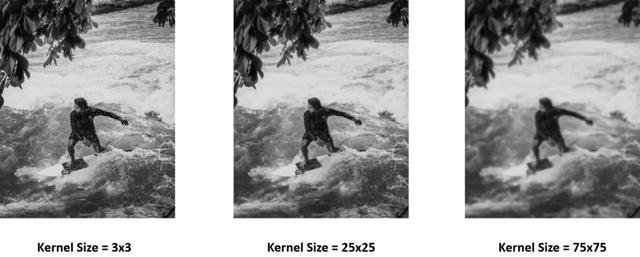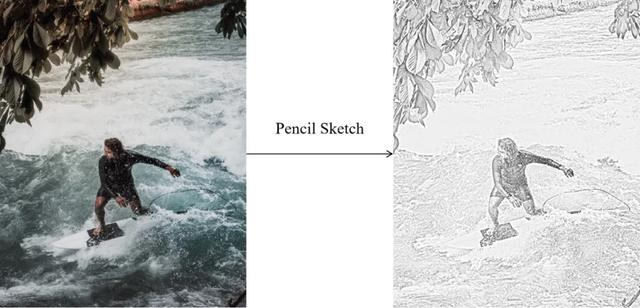```#convert the image into grayscale image
gray = cv2.cvtColor(img, cv2.COLOR_BGR2GRAY)

#Blur the grayscale image with median blur
gray_blur = cv2.medianBlur(gray, 3)

#Apply adaptive thresholding to detect edges

#Sharpen the image
color = cv2.detailEnhance(img, sigma_s=5, sigma_r=0.5)

#Merge the colors of same images using "edges" as a mask• sigma_s：控制着邻域的大小，该邻域的大小将被加权以替换图像中的像素值。值越高，邻域越大。这样可以使图像更平滑。

• sigma_r：如果要在平滑图像时保留边缘，这很重要。较小的值只会产生非常相似的颜色进行平均（即平滑），而相差很大的颜色将保持不变。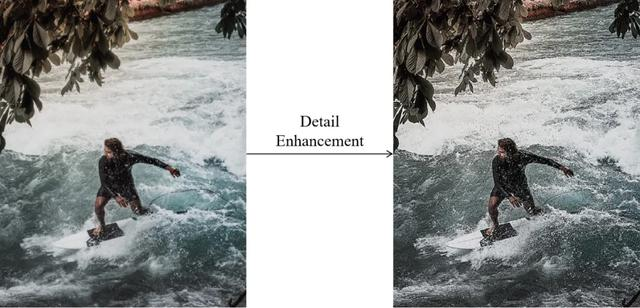```#convert the image into grayscale image
gray = cv2.cvtColor(img, cv2.COLOR_BGR2GRAY)

#Blur the grayscale image with median blur
gray_blur = cv2.medianBlur(gray, 3)

#Apply adaptive thresholding to detect edges

#Sharpen the image
color = cv2.detailEnhance(img, sigma_s=5, sigma_r=0.5)

#Merge the colors of same images using "edges" as a mask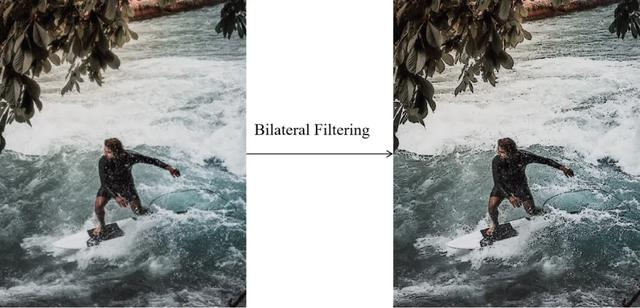```#Convert the image into grayscale image
gray = cv2.cvtColor(img, cv2.COLOR_BGR2GRAY)

#Blur the grayscale image using median blur
gray = cv2.medianBlur(gray, 25)

#Detect edges with Laplacian
edges = cv2.Laplacian(gray, -1, ksize=3)

#Invert the edges
edges_inv = 255-edges

#Create a pencil edge sketch
dummy, cartoon = cv2.threshold(edges_inv, 150, 255, cv2.THRESH_BINARY)```

Laplacian滤波器的工作是，将通过对象内部的灰度级和图像背景强度来突出对象的边缘。以下是拉普拉斯滤波器应用结果。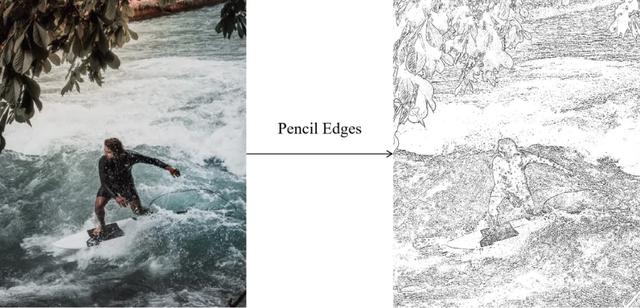Pencil Edges滤镜实现示例

```gray = cv2.cvtColor(img, cv2.COLOR_BGR2GRAY)
scale_val = st.slider('Tune the brightness of your sketch (the higher the value, the brighter your sketch)', 0.0, 300.0, 250.0)
kernel = st.slider('Tune the boldness of the edges of your sketch (the higher the value, the bolder the edges)', 1, 99, 25, step=2)
gray_blur = cv2.GaussianBlur(gray, (kernel, kernel), 0)
cartoon = cv2.divide(gray, gray_blur, scale= scale_val)```

```import cv2
import streamlit as st
import numpy as np
from PIL import Image

def cartoonization (img, cartoon):

gray = cv2.cvtColor(img, cv2.COLOR_BGR2GRAY)

if cartoon == "Pencil Sketch":

value = st.sidebar.slider('Tune the brightness of your sketch (the higher the value, the brighter your sketch)', 0.0, 300.0, 250.0)
kernel = st.sidebar.slider('Tune the boldness of the edges of your sketch (the higher the value, the bolder the edges)', 1, 99, 25, step=2)

gray_blur = cv2.GaussianBlur(gray, (kernel, kernel), 0)

cartoon = cv2.divide(gray, gray_blur, scale=value)

if cartoon == "Detail Enhancement":

smooth = st.sidebar.slider('Tune the smoothness level of the image (the higher the value, the smoother the image)', 3, 99, 5, step=2)
kernel = st.sidebar.slider('Tune the sharpness of the image (the lower the value, the sharper it is)', 1, 21, 3, step =2)
edge_preserve = st.sidebar.slider('Tune the color averaging effects (low: only similar colors will be smoothed, high: dissimilar color will be smoothed)', 0.0, 1.0, 0.5)

gray = cv2.medianBlur(gray, kernel)
cv2.THRESH_BINARY, 9, 9)

color = cv2.detailEnhance(img, sigma_s=smooth, sigma_r=edge_preserve)

if cartoon == "Pencil Edges":

kernel = st.sidebar.slider('Tune the sharpness of the sketch (the lower the value, the sharper it is)', 1, 99, 25, step=2)
laplacian_filter = st.sidebar.slider('Tune the edge detection power (the higher the value, the more powerful it is)', 3, 9, 3, step =2)
noise_reduction = st.sidebar.slider('Tune the noise effects of your sketch (the higher the value, the noisier it is)', 10, 255, 150)

gray = cv2.medianBlur(gray, kernel)
edges = cv2.Laplacian(gray, -1, ksize=laplacian_filter)

edges_inv = 255-edges

dummy, cartoon = cv2.threshold(edges_inv, noise_reduction, 255, cv2.THRESH_BINARY)

if cartoon == "Bilateral Filter":

smooth = st.sidebar.slider('Tune the smoothness level of the image (the higher the value, the smoother the image)', 3, 99, 5, step=2)
kernel = st.sidebar.slider('Tune the sharpness of the image (the lower the value, the sharper it is)', 1, 21, 3, step =2)
edge_preserve = st.sidebar.slider('Tune the color averaging effects (low: only similar colors will be smoothed, high: dissimilar color will be smoothed)', 1, 100, 50)

gray = cv2.medianBlur(gray, kernel)
cv2.THRESH_BINARY, 9, 9)

color = cv2.bilateralFilter(img, smooth, edge_preserve, smooth)

return cartoon

###############################################################################

st.write("""

"""
)

st.write("This is an app to turn your photos into cartoon")

if file is None:
st.text("You haven't uploaded an image file")
else:
image = Image.open(file)
img = np.array(image)

option = st.sidebar.selectbox(
'Which cartoon filters would you like to apply?',
('Pencil Sketch', 'Detail Enhancement', 'Pencil Edges', 'Bilateral Filter'))

st.image(image, use_column_width=True)

cartoon = cartoonization(img, option)

st.image(cartoon, use_column_width=True)```

`streamlit run your_app_name.py`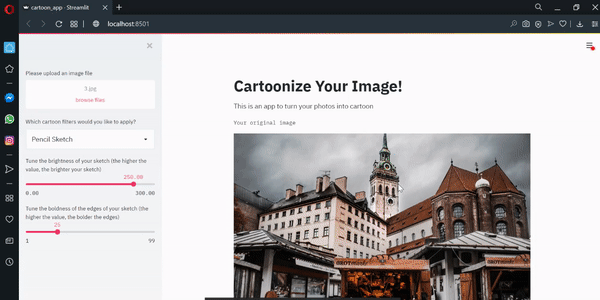• requirements.txt：这是文本文件，用于告诉Heroku构建Web应用程序需要哪些依赖项。因为在我们的web应用程序，我们使用四种不同的库：opencv，numpy，Pillow，和streamlit，那么我们就可以写所有这些库及其版本为requirements.txt的

```opencv-python==4.3.0.36
streamlit==0.63.0
Pillow==7.0.0
numpy==1.18.1```

• setup.sh：这是用于在Heroku上设置配置的文件。为此setup.sh文件编写以下内容。

```mkdir -p ~/.streamlit/
echo "\
[server]\n\
port = \$PORT\n\
enableCORS = false\n\
\n\
" > ~/.streamlit/config.toml```

• Procfile：这是告诉Heroku哪些文件以及应如何执行文件的文件。为Procfile编写以下内容。

`web: sh setup.sh && streamlit run cartoon_app.py`

• Aptfile：这是Heroku 构建包的文件，以使OpenCV能够在Heroku中运行。为Aptfile编写以下内容。

```libsm6
libxrender1
libfontconfig1
libice6```

`heroku git:remote -a your-app-name`

`heroku create --buildpack https://github.com/heroku/heroku-buildpack-apt.git`

```git init
git push heroku master```

OK